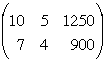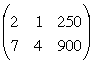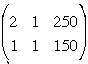SEARCH HOMEMath Central Quandaries & QueriesQuestion from sandhiya, a student: one group of people purchased 10 hotdogs and 5 soft drinks at a cost of $12.50.asecond group bought 7 hotdogs and 4 soft drinks at a cost of$9.00 find the cost of a single hot and a single soft drink.(using the row echelon form to solve)Hi Sandhiya,

I can help get you started.

First I am going to write the cost on cents rather than dollars so that I can work with integers. Let h be the cost of a hotdog in cents and s be the cost of a soft drink in cents. Then for the first group

10 h + 5 s = 1250

and for the second group

7 h + 4 s = 900.

The augmented matrix for this system of linear equations is thenThe first thing I notice is that the first row has a common factor of 5 so multiply the first row by 1/5Multiply the first row by -3 add add it to the second rowCan you complete the problem now?

PennyMath Central is supported by the University of Regina and The Pacific Institute for the Mathematical Sciences.